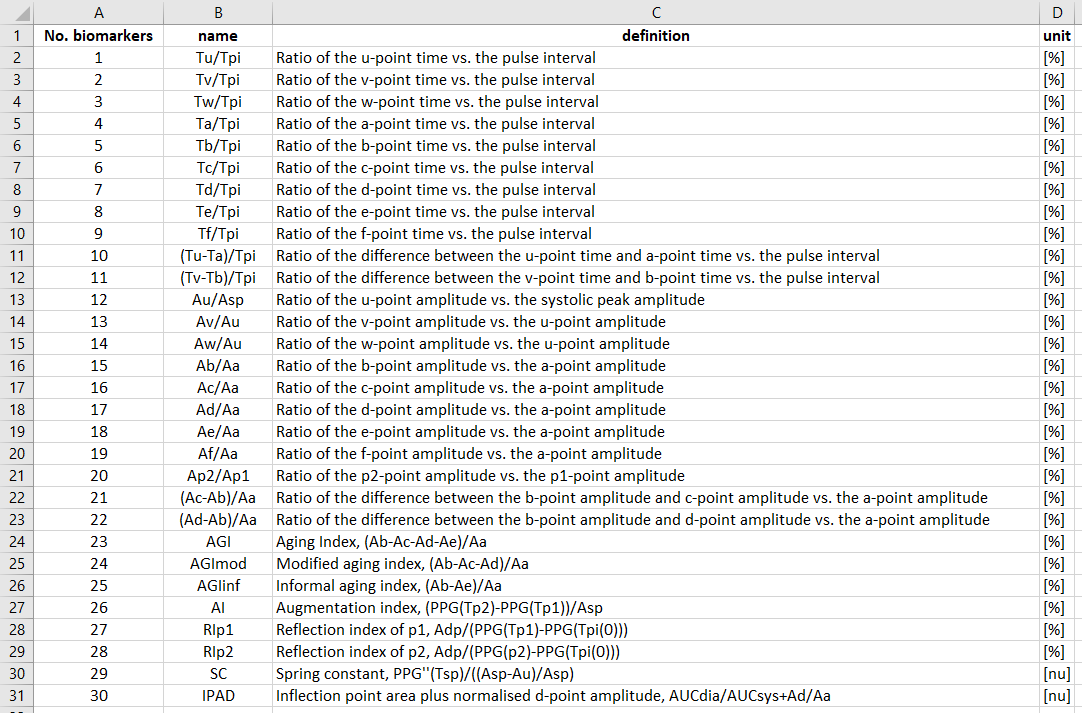# Comprehensive PPG Analysis

Colab Notebook

In this tutorial we will learn how to extract biomarkers from a photoplethysmogram (PPG) signal.

Our objectives are to:

• Detect the standard fiducial points on PPG pulse waves

• Calculate pulse wave biomarkers from the fiducial points

• Saving data in different data format

## Setup

### Import Python packages:

• Install the pyPPG toolbox for PPG analysis

```pip install pyPPG==1.0.41
```
• Import required components from pyPPG

```from pyPPG import PPG, Fiducials, Biomarkers
from pyPPG.datahandling import load_data, plot_fiducials, save_data
import pyPPG.preproc as PP
import pyPPG.fiducials as FP
import pyPPG.biomarkers as BM
import pyPPG.ppg_sqi as SQI
```
• Import other packages

```import numpy as np
import sys
import json
import pandas as pd
```

### Setup input parameters:

The following input parameters are inputs to the pyPPG.example module (see the documentation for further details).

```data_path = "Sample_PPG_MAT_125Hz.mat" # the path of the file containing the PPG signal to be analysed
start_sig = 0 # the first sample of the signal to be analysed
end_sig = -1 # the last sample of the signal to be analysed (here a value of '-1' indicates the last sample)
savingfolder = 'temp_dir'
savingformat = 'csv'
```

```# Load the raw PPG signal
signal = load_data(data_path=data_path, start_sig=start_sig, end_sig=end_sig, use_tk=False)
signal.v = signal.v [0:20*signal.fs] # 20 second long signal to be analysed
```

### Plot the raw PPG signal:

```# import plotting package
from matplotlib import pyplot as plt

# setup figure
fig, ax = plt.subplots()

# create time vector
t = np.arange(0, len(signal.v))/signal.fs

# plot raw PPG signal
ax.plot(t, signal.v, color = 'blue')
ax.set(xlabel = 'Time (s)', ylabel = 'raw PPG')

# show plot
plt.show()
```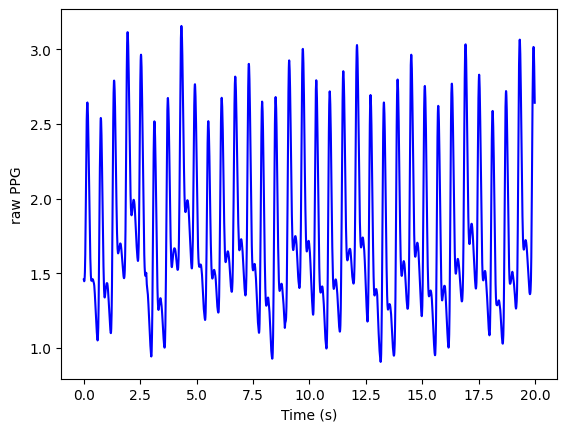## PPG signal processing

### Prepare the PPG data:

Filter the PPG signal and obtain first, second and third derivatives (vpg, apg, and jpg respectively).

```signal.filtering = True # whether or not to filter the PPG signal
signal.fL=0.5000001 # Lower cutoff frequency (Hz)
signal.fH=12 # Upper cutoff frequency (Hz)
signal.order=4 # Filter order
signal.sm_wins={'ppg':50,'vpg':10,'apg':10,'jpg':10} # smoothing windows in millisecond for the PPG, PPG', PPG", and PPG'"

prep = PP.Preprocess(fL=signal.fL, fH=signal.fH, order=signal.order, sm_wins=signal.sm_wins)
signal.ppg, signal.vpg, signal.apg, signal.jpg = prep.get_signals(s=signal)
```

Plot the derived signals

```# setup figure
fig, (ax1,ax2,ax3,ax4) = plt.subplots(4, 1, sharex = True, sharey = False)

# create time vector
t = np.arange(0, len(signal.ppg))/signal.fs

# plot filtered PPG signal
ax1.plot(t, signal.ppg)
ax1.set(xlabel = '', ylabel = 'PPG')

# plot first derivative
ax2.plot(t, signal.vpg)
ax2.set(xlabel = '', ylabel = 'PPG\'')

# plot second derivative
ax3.plot(t, signal.apg)
ax3.set(xlabel = '', ylabel = 'PPG\'\'')

# plot third derivative
ax4.plot(t, signal.jpg)
ax4.set(xlabel = 'Time (s)', ylabel = 'PPG\'\'\'')

# show plot
plt.show()
```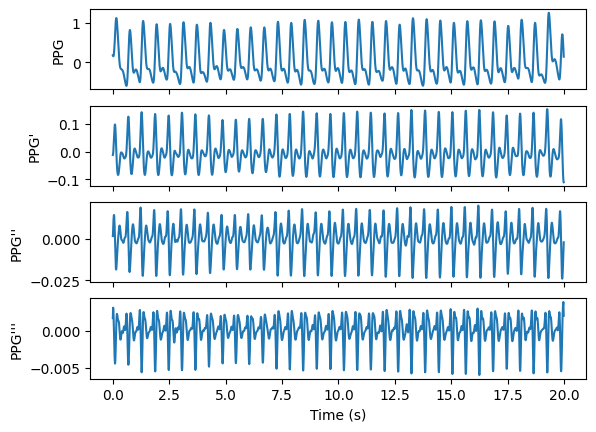Store the derived signals in a class

```# Initialise the correction for fiducial points
corr_on = ['on', 'dn', 'dp', 'v', 'w', 'f']
correction=pd.DataFrame()
correction.loc[0, corr_on] = True
signal.correction=correction

# Create a PPG class
s = PPG(signal)
```

### Identify fiducial points:

Initialise the fiducials package

```fpex = FP.FpCollection(s=s)
```

Extract fiducial points

```fiducials = fpex.get_fiducials(s=s)
```

Display the results

```print("Fiducial points:\n",fiducials + s.start_sig) # here the starting sample is added so that the results are relative to the start of the original signal (rather than the start of the analysed segment)
```

### Plot fiducial points:

```# Create a fiducials class
fp = Fiducials(fp=fiducials)

# Plot fiducial points
plot_fiducials(s, fp, savingfolder, legend_fontsize=12)
```
PPG fiducial points## Calculate PPG SQI:

```# Get PPG SQI
ppgSQI = round(np.mean(SQI.get_ppgSQI(ppg=s.ppg, fs=s.fs, annotation=fp.sp)) * 100, 2)
print('Mean PPG SQI: ', ppgSQI, '%')
```

## Calculate PPG biomarkers:

```# Init the biomarkers package
bmex = BM.BmCollection(s=s, fp=fp)

# Extract biomarkers
bm_defs, bm_vals, bm_stats = bmex.get_biomarkers()
tmp_keys=bm_stats.keys()
print('Statistics of the biomarkers:')
for i in tmp_keys: print(i,'\n',bm_stats[i])

# Create a biomarkers class
bm = Biomarkers(bm_defs=bm_defs, bm_vals=bm_vals, bm_stats=bm_stats)
```

## Save PPG data:

```# Save PPG struct, fiducial points, biomarkers
fp_new = Fiducials(fp.get_fp() + s.start_sig) # here the starting sample is added so that the results are relative to the start of the original signal (rather than the start of the analysed segment)
save_data(s=s, fp=fp_new, bm=bm, savingformat=savingformat, savingfolder=savingfolder)
```
Extracted fiducial points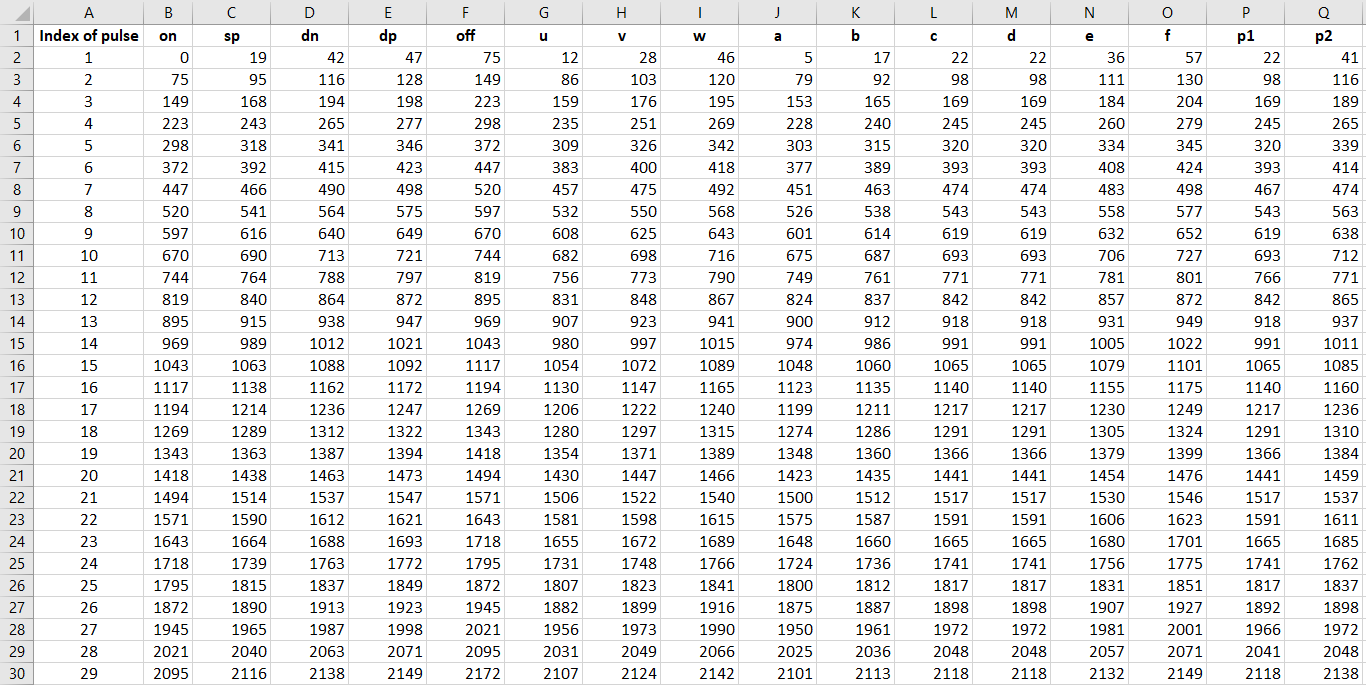Extracted biomarkers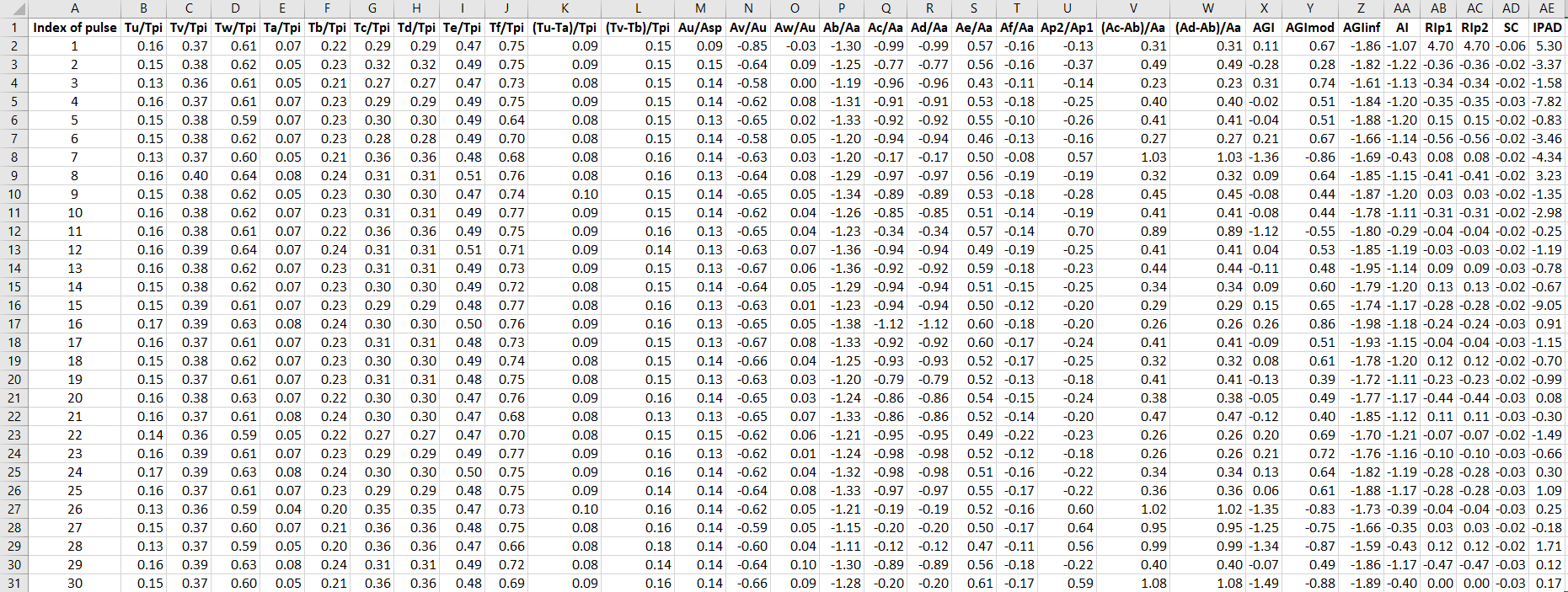Biomarkers statistics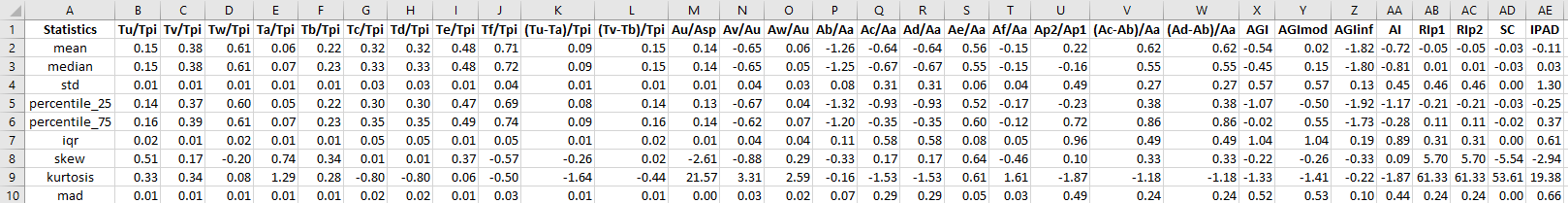Biomarkers definitions